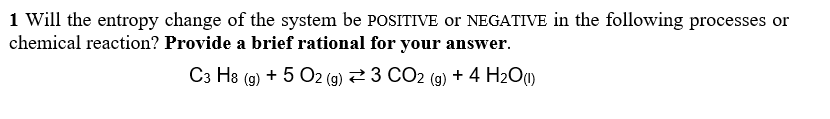# 1 Will the entropy change of the system be POSITIVE or NEGATIVE in the following processes orchemical reaction? Provide a brief rational for your answerC3 H8 (g) + 5 O2 (g):3 CO2 (g) + 4 H2O(l)

Question
13 viewshelp_outlineImage Transcriptionclose1 Will the entropy change of the system be POSITIVE or NEGATIVE in the following processes or chemical reaction? Provide a brief rational for your answer C3 H8 (g) + 5 O2 (g) :3 CO2 (g) + 4 H2O(l) fullscreen
check_circle

Step 1

An increase in entropy results from an increase in disorder.

Entropy is an extensive property (a function of number of moles).

Step 2

The stoichiometric coefficient of product minus coefficient of reactant used to predicts the entropy change of the system.

Step 3

Number of moles on the product side is 7(3+4) and the number of moles of reactant is 6(1+5)

∆S0 is 7 - (6) = 1

Six moles of reactant gives 7 mole...

### Want to see the full answer?

See Solution

#### Want to see this answer and more?

Solutions are written by subject experts who are available 24/7. Questions are typically answered within 1 hour.*

See Solution
*Response times may vary by subject and question.
Tagged in

### Chemistry